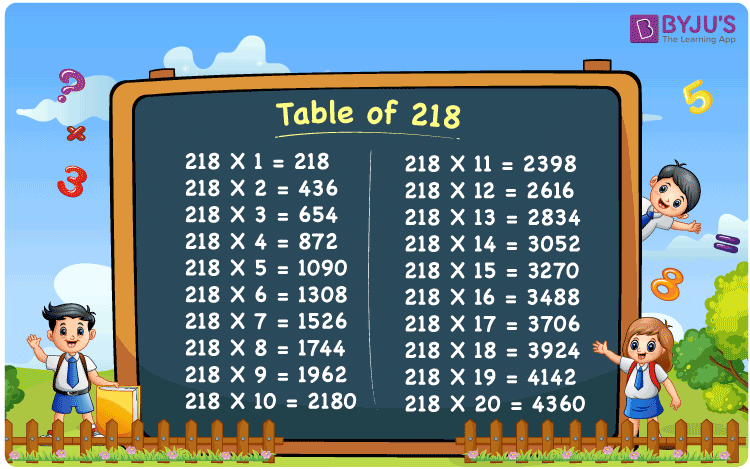Checkout JEE MAINS 2022 Question Paper Analysis : Checkout JEE MAINS 2022 Question Paper Analysis :

# Table of 218

The table of 218 is given here using two different mathematical operations, such as addition and multiplication. As we know, maths tables are a useful tool for solving numerical problems accurately. Thus, learning the table of 218 will help you to simplify the numerical expressions involving the multiples of 218. Students can also download the PDF of the table of 218 given below.

## Table of 218 Chart## What is the 218 Times Table?

The 218 times table can be generated using the repeated addition of 218 or by multiplying 218 by numbers 1, 2, 3, and so on. This can be observed from the table given below.

 Using multiplication Using repeated addition 218 × 1 = 218 218 218 × 2 = 436 218 + 218 = 436 218 × 3 = 654 218 + 218 + 218 = 654 218 × 4 = 872 218 + 218 + 218 + 218 = 872 218 × 5 = 1090 218 + 218 + 218 + 218 + 218 = 1090 218 × 6 = 1308 218 + 218 + 218 + 218 + 218 + 218 = 1308 218 × 7 = 1526 218 + 218 + 218 + 218 + 218 + 218 + 218 = 1526 218 × 8 = 1744 218 + 218 + 218 + 218 + 218 + 218 + 218 + 218 = 1744 218 × 9 = 1962 218 + 218 + 218 + 218 + 218 + 218 + 218 + 218 + 218 = 1962 218 × 10 = 2180 218 + 218 + 218 + 218 + 218 + 218 + 218 + 218 + 218 + 218 = 2180

## Multiplication Table of 218

Go through the multiplication table 218 given here using the multiplication of numbers.

 218 × 1 = 218 218 × 2 = 436 218 × 3 = 654 218 × 4 = 872 218 × 5 = 1090 218 × 6 = 1308 218 × 7 = 1526 218 × 8 = 1744 218 × 9 = 1962 218 × 10 = 2180 218 × 11 = 2398 218 × 12 = 2616 218 × 13 = 2834 218 × 14 = 3052 218 × 15 = 3270 218 × 16 = 3488 218 × 17 = 3706 218 × 18 = 3924 218 × 19 = 4142 218 × 20 = 4360

## Solved Examples

Example 1:

Suppose one park has 218 trees; how many trees do seven parks have?

Solution:

Given,

Number of trees in a park = 218

Number of parks = 7

Using the table of 218, 218 × 7 = 1526.

Thus, the total number of trees in 7 parks = 218 × 7 = 1526.

Example 2:

A retailer has 218 boxes of 16 cells each. How many cells do all the boxes have in all?

Solution:

Number of boxes = 218

Number of cells in each box = 16

Using the table of 218, 218 × 16 = 3488.

Therefore, the total number of cells in all the boxes together = 218 × 16 = 3488.

## Frequently Asked Questions on Table of 218

### What is the table of 218?

The table of 218 is given as: 218 × 1 = 218 218 × 2 = 436 218 × 3 = 654 218 × 4 = 872 218 × 5 = 1090 218 × 6 = 1308, etc.

### How do you calculate the value of 218 times 5?

We can calculate the value of 218 times 5 either by multiplying 218 by 5 or by repeatedly adding 218 for 5 times. That means, 218 times 5 = 218 × 5 = 1090 Or 218 + 218 + 218 + 218 + 218 = 1090.

### In which table does 218 come?

In the table of 2 or in the table of 109, we can get the number 218 as the result, i.e. 2 × 109 or 109 × 2 = 218.

### Is 218 in the 8 times table?

No, 218 does not appear in the 8 times table, from the results of the 8 times table, we can verify the same, i.e. 8, 16, 24, 32, 40, 48, 56, 64, 72, 80, 88, 96, 104, 112, 120, 128, 136, 144, 152, 160, 168, 176, 184, 192, 200, 208, 216, 224, 232,…